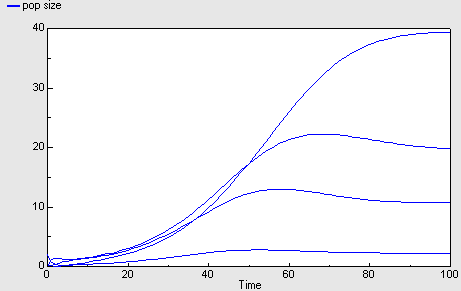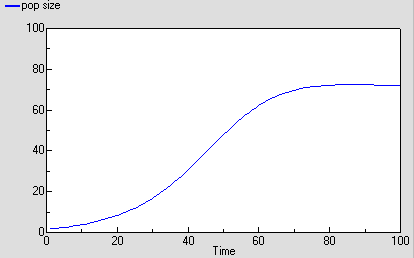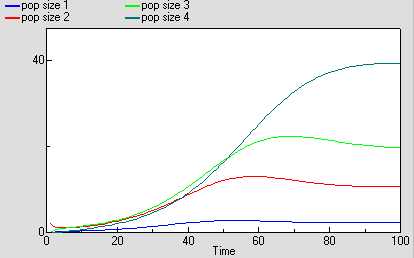# Age-class modelling

Modelling a population in terms of the number of individuals in different age-classes

## Age-class model of population dynamics, using a multiple-instance submodel and an association submodel

ModelId:
ageclass6
SimileVersion:
5.9

This models a population of animals in terms of four age-classes. For each age-class, we use a compartment (state variable) to represent the number of individuals in that class. This version uses a four-instance submodel to represent the four age-classes. The ageing flow (from one class to the next) thus has to be handled by transferring the value for the outflow from each class to be assigned to the inflow for the next class.

Equations:

Equations in Desktop

births = sum([these_births])
pop size = sum([pop_size])

Equations in Age class

pop size: initial value = element([2,0,0,0],index(1))

ageing in = sum({ageing_next})
Where: {ageing_next}=../Next class/ageing (to Age class in next)

ageing out = element([1,0.2,0.1,0],index(1))*pop_size

births = if index(1)==1 then births else 0

deaths = m*pop_size

class = index(1)

m = element([0.05,0.01,0.01,0.05],index(1))

r = element([0,0.05,0.2,0.1],index(1))*(1-total_pop_size/100)

these births = r*pop_size

Equations in Next class

condition = class_next==class_this+1
Where: class_this=../Age class/class (from Age class in this) class_next=../Age class/class (from Age class in next)

ageing = ageing_out_this
Where: ageing_out_this=../Age class/ageing out (from Age class in this)

here: ageing_out_this=../Age class/ageing out (from Age class in this)/

Results:## Age-class model of population dynamics, using multiple-instance submodel

ModelId:
ageclass5
SimileVersion:
5.9

This models a population of animals in terms of four age-classes. For each age-class, we use a compartment (state variable) to represent the number of individuals in that class.

Equations:

Equations in Desktop

births = sum([these_births])
pop size = sum([pop_size])
ageing = [ageing_out]

Equations in Age class
pop size: initial value = element([2,0,0,0],index(1))
births = if index(1) == 1 then births else 0
ageing out = element([1,0.2,0.1,0],index(1))*pop_size
deaths = m*pop_size
ageing in = if index(1)>1 then element([ageing],index(1)-1)else 0
m = element([0.05,0.01,0.01,0.05],index(1))
r = element([0,0.05,0.2,0.1],index(1))*(1-pop_size/100)
these births= r*pop_size

Results:Model tags:

## Age-class model of population dynamics, one compartment per age class

ModelId:
ageclass4
SimileVersion:
5.9

This models a population of animals in terms of four age-classes. For each age-class, we use a compartment (state variable) to represent the number of individuals in that class.

Equations:

Compartments

pop_size_1: initial value = 2
pop size_2: initial value = 0
pop size_3: initial value = 0
pop size_4: initial value = 0

Flows
ageing_1 = pop_size_1
ageing_2 = 0.2*pop_size_2
ageing_3 = 0.1*pop_size_3
births = births_2+births_3+births_4
deaths_1 = m1*pop_size_1
deaths_2 = m2*pop_size_2
deaths_3 = m3*pop_size_3
deaths_4 = m4*pop_size_4

Parameters
m1 = 0.05
m2 = 0.01
m3 = 0.01
m4 = 0.05

Intermediate variables
births_2 = r2*pop_size_2
births_3 = r3*pop_size_3
births_4 = r4*pop_size_4
pop size = pop_size_1+pop_size_2+pop_size_3+pop_size_4
r2 = 0.05*(1-pop_size/100)
r3 = 0.2*(1-pop_size/100)

Results:Model tags: